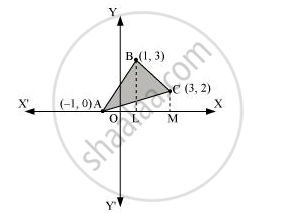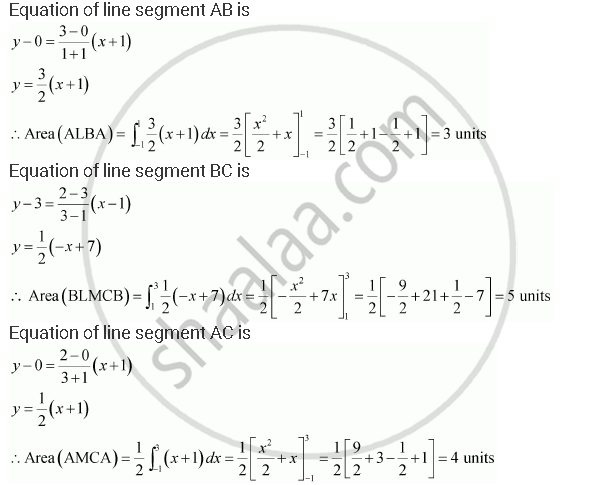Share

# Using Integration Finds the Area of the Region Bounded by the Triangle Whose Vertices Are (–1, 0), (1, 3) and (3, 2). - CBSE (Science) Class 12 - Mathematics

#### Question

Using integration finds the area of the region bounded by the triangle whose vertices are (–1, 0), (1, 3) and (3, 2).

#### Solution

BL and CM are drawn perpendicular to x-axis.

It can be observed in the following figure that,

Area (ΔACB) = Area (ALBA) + Area (BLMCB) – Area (AMCA) … (1)Therefore, from equation (1), we obtain

Area (ΔABC) = (3 + 5 – 4) = 4 units

Is there an error in this question or solution?

#### APPEARS IN

NCERT Solution for Mathematics Textbook for Class 12 (2018 to Current)
Chapter 8: Application of Integrals
Q: 4 | Page no. 371

#### Video TutorialsVIEW ALL 

Solution Using Integration Finds the Area of the Region Bounded by the Triangle Whose Vertices Are (–1, 0), (1, 3) and (3, 2). Concept: Area Between Two Curves.
S